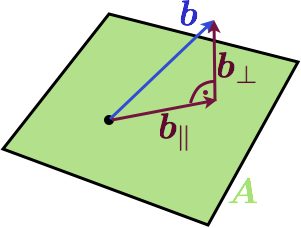Your browser (Internet Explorer 6) is out of date. It has known security flaws and may not display all features of this and other websites. Learn how to update your browser.
X
The Linear Algebra View on Least Squares

# A Linear Algebra View on Least Squares

Last time, we introduce the least squares problem and took a direct approach to solving it. Basically we just minimized the $y$-distance of a model to the data by varying the parameters of the model. Today, we find a completely different, but very neat interpretation.

Suppose we have the following problem: There is a set of linear equations without a solution, e.q. a matrix $\mat{A}$ and a vector $\vec{b}$ so that no vector $\vec{x}$ exists that would solve the equation.

\begin{equation*}\mat{A} \vec{x} = \vec{b}.\end{equation*}

Bummer. What does that actually mean though? If we use every possible value of $\vec{x}$ in this equation, we get essentially all linear combinations of the column vectors of $A$. That is a span of vectors. That is a subspace. So, $\vec{b}$ is not in our subspace, otherwise we would find an $\vec{x}$ that would solve our linear equation.

Here is a visualization in 3D when A is a two dimensional matrix:The column space of the matrix is a plane and $b$ is not in the plane. A natural question now is: what is the vector in the column space of $\mat{A}$ that is the best approximation to $b$. But what is a good approximation? Well, how about the Euclidian distance between the endpoints of the vectors. If we choose this criteria, there is a simple solution: the projection of $\vec{b}$ to $\mat{A}$ will give the best approximation. Here is another image:We have decomposed $\vec{b}$ into its best approximation in $\mat{A}$ which we call $\vec{b_\parallel}$ and a component that is orthogonal to it called $\vec{b_}\perp$. At the end of the day, we are interested in a best approximated solution to our original equation, so we are not really interested in finding $\vec{b}_\parallel$, but rather an $\vec{x^*}$ so that

\begin{equation*}\mat{A} \vec{x}^* = \vec{b}_\parallel\end{equation*}

Lets look for an equation for this $\vec{x}^*$. We will start out by using that the two components of $\vec{b}$ are perpendicular to each other. And we will also express $\vec{b}_\perp$ through vector addition.

\begin{align*}0 &= \vec{b}_\parallel ^T \vec{b}_\perp \\ &= (\mat{A} \vec{x}^*)^T \vec{b}_\perp \\ &= (\mat{A} \vec{x}^*)^T (\vec{b} - \mat{A} \vec{x}^*) \\ &= \vec{x}^{* T} \mat{A}^T (\vec{b} - \mat{A} \vec{x}^*) \\ &= \vec{x}^{* T} ( \mat{A}^T \vec{b} - \mat{A}^T \vec{A} \vec{x}^*)\end{align*}

Since $\vec{x}^*$ can't be zero, the term in parenthesis must be. This directly yields the least squares formula again:

\begin{equation*}\vec{x}^* = (\mat{A}^T \mat{A})^{-1} \mat{A}^T \vec{b}\end{equation*}

## Conclusion

We did the exact same thing as in the last post, but this time we took another approach and quite a different world view on the problem. The least squares solution came up very naturally here and with a very simple geometric interpretation (at least in the 3d case, the approach is also valid for other dimensions though).

The last iteration of this series will take a look at least squares in statistical models.